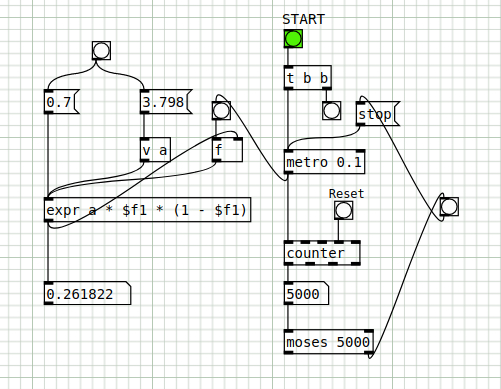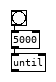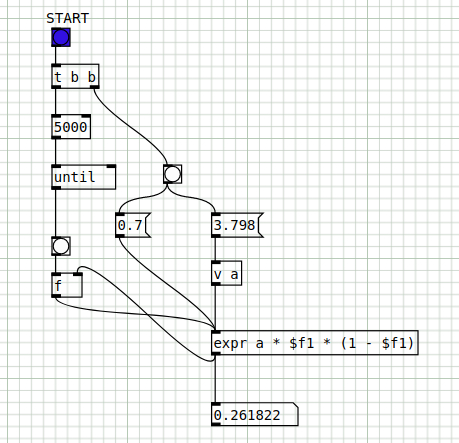• ### Recursion

Hi all,

in this patch I would like to do 5000 iterations of a recursive function.
I used the objects [metro], [counter] and [moses]. Is this the correct way to do this?
In particular, I would like it to be done as quickly as possible.

Note: 0.7 is the initial value, 3.798 is the constant "a" . Both values ​​are entered automatically before running the iterations.recursive.pd
a.

• Posts 7 | Views 312
• @atux [until] is for recursion and what you should use anytime you need it done as fast as possible. Much simpler as well. Give the helpfile for [until] a read.• Hi,

this simplifies a lot and that's exactly what I was looking for, the immediate calculation.
Thank you.

EDIT: I just have to figure out how, using [until], everything stays in sync (in fact it works fine). The output value must be stored and then re-entered, and this happens.recursive2.pd

• @atux Just curious, what are you using this calculation for?

• @jameslo said:

@atux Just curious, what are you using this calculation for?

...for Bifurcation diagram (Logistic map).

a.

• @atux Interesting! I understand why you have to start the recursion with a non-zero input, but why did you choose 0.7?

• @jameslo said:

@atux Interesting! I understand why you have to start the recursion with a non-zero input, but why did you choose 0.7?

For that value of constant a, any initial value between 0 and 1 gives interesting results.

Posts 7 | Views 312
Internal error.

Oops! Looks like something went wrong!